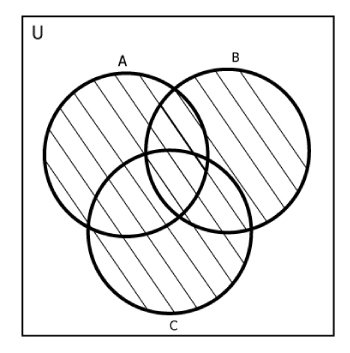# Python Set union()

The union() method returns a new set with distinct elements from all the sets.

The union of two or more sets is the set of all distinct elements present in all the sets. For example:

```A = {1, 2}
B = {2, 3, 4}
C = {5}

Then,
A∪B = B∪A ={1, 2, 3, 4}
A∪C = C∪A ={1, 2, 5}
B∪C = C∪B ={2, 3, 4, 5}

A∪B∪C = {1, 2, 3, 4, 5}```The syntax of union() is:

`A.union(*other_sets)`

## union() Parameters

In Python, union() allows arbitrary number of arguments.

Note: * is not part of the syntax. It is used to indicate that the method allows arbitrary number of arguments.

## Return Value from union()

The union() method returns the union of set A with all the sets (passed as an argument).

If argument is not passed to union(), it returns a shallow copy the set.

## Example 1: How union() works?

```A = {'a', 'c', 'd'}
B = {'c', 'd', 2 }
C= {1, 2, 3}

print('A U B =', A.union(B))
print('B U C =', B.union(C))

print('A U B U C =', A.union(B, C))

print('A.union() = ', A.union())```

When you run the program, the output will be:

```A U B = {2, 'a', 'd', 'c'}
B U C = {1, 2, 3, 'd', 'c'}
A U B U C = {1, 2, 3, 'a', 'd', 'c'}
A.union() =  {'a', 'd', 'c'}```

You can also find the union of sets using | operator.

## Example 2: Set Union Using | Operator

```A = {'a', 'c', 'd'}
B = {'c', 'd', 2 }
C= {1, 2, 3}

print('A U B =', A| B)
print('B U C =', B | C)

print('A U B U C =', A | B | C)
```

When you run the program, the output will be:

```A U B = {2, 'a', 'c', 'd'}
B U C = {1, 2, 3, 'c', 'd'}
A U B U C = {1, 2, 3, 'a', 'c', 'd'}```